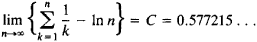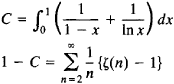# Euler's Constant

(redirected from Euler constant)

## Euler's constant

[′ȯi·lərz ¦kän·stənt]
(mathematics)
The limit as n approaches infinity, of 1 + 1/2 + 1/3 + ⋯ + 1/ n- ln n, equal to approximately 0.5772. Denoted γ. Also known as Mascheroni's constant.
McGraw-Hill Dictionary of Scientific & Technical Terms, 6E, Copyright © 2003 by The McGraw-Hill Companies, Inc.
The following article is from The Great Soviet Encyclopedia (1979). It might be outdated or ideologically biased.

## Euler’s Constant

(or Mascheroni’s constant), the limitwhich was considered by L. Euler in 1740. Euler gave a number of representations for C in the form of series and integrals; for example,where ζ(s) is the zeta function. Euler’s constant is encountered in the theory of various classes of special functions, such as the gamma function. It remains unknown whether Euler’s constant is an irrational number.

Mentioned in ?
References in periodicals archive ?
where [gamma] is the Euler constant, [LAMBDA](n) is the Mangoldt function, and [MATHEMATICAL EXPRESSION NOT REPRODUCIBLE IN ASCII.] denotes the
Here, [??] denotes the congruence zeta function of the direct product of n - 1 copies of the multiplicative group scheme [G.sub.m] over [F.sub.p], and [gamma](p) is the p-analogue of the Euler constant (2) [gamma], which is defined by
where B = [summation over (p)] 1/[p.sup.2], D = [summation over [p]] ln p/[p.sup.2], [gamma] is the Euler constant, and [summation over (p)] denotes the summation over all primes.
where A = [gamma] + [[SIMGA].sub.p]p (ln(1-1/p) + 1/p - 1] be a constant, [[SIMGA].sub.p] denotes the summation over all primes, and [gamma] be the Euler constant.
where [gamma] is the Euler constant, [epsilon] denotes any fixed positive number.
[gamma] is the Euler constant. [B.sub.1], [B.sub.2], [B.sub.3], [C.sub.1], [C.sub.2], [D.sub.1], [D.sub.2], [D.sub.3], [D.sub.4] are constants.
where c is a constant which depends on q and l, [gamma] is the Euler constant.
where [gamma] is the Euler constant, " denotes any fixed positive number.
where [gamma] is the Euler constant, denotes any fixed positive number.
where A = [gamma] + [summation over (p)](log(1 [x.sup.-1] 1/p) + 1/p), [gamma] is the Euler constant, a and b are two computable
where B = 4C - 1/2 + 3/2 log 2- 315[zeta](3)/[6[pi].sup.4], C is Euler constant, d(n) be the Dirichlet divisor function.

Site: Follow: Share:
Open / Close